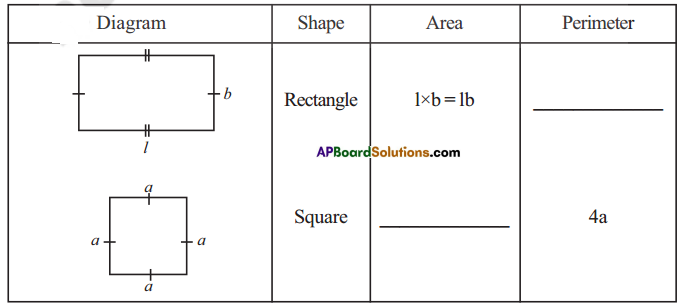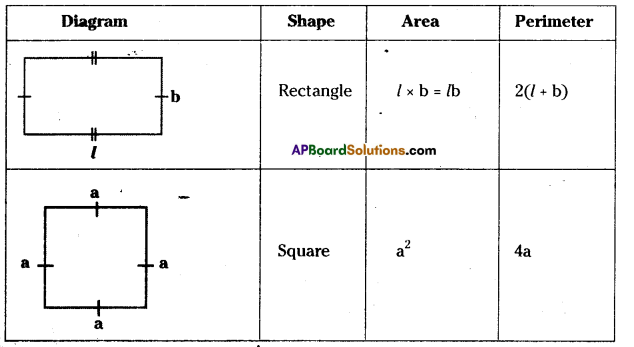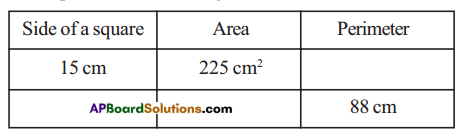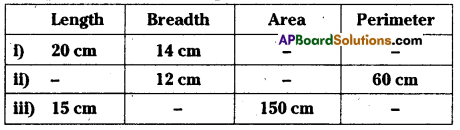# HSSlive: Plus One & Plus Two Notes & Solutions for Kerala State Board

## AP Board Class 7 Maths Chapter 13 Area and Perimeter Ex 1 Textbook Solutions PDF: Download Andhra Pradesh Board STD 7th Maths Chapter 13 Area and Perimeter Ex 1 Book AnswersAP Board Class 7 Maths Chapter 13 Area and Perimeter Ex 1 Textbook Solutions PDF: Download Andhra Pradesh Board STD 7th Maths Chapter 13 Area and Perimeter Ex 1 Book Answers

## Andhra Pradesh State Board Class 7th Maths Chapter 13 Area and Perimeter Ex 1 Books Solutions

 Board AP Board Materials Textbook Solutions/Guide Format DOC/PDF Class 7th Subject Maths Chapters Maths Chapter 13 Area and Perimeter Ex 1 Provider Hsslive

2. Click on the Andhra Pradesh Board Class 7th Maths Chapter 13 Area and Perimeter Ex 1 Answers.
3. Look for your Andhra Pradesh Board STD 7th Maths Chapter 13 Area and Perimeter Ex 1 Textbooks PDF.
4. Now download or read the Andhra Pradesh Board Class 7th Maths Chapter 13 Area and Perimeter Ex 1 Textbook Solutions for PDF Free.

## AP Board Class 7th Maths Chapter 13 Area and Perimeter Ex 1 Textbooks Solutions with Answer PDF Download

Find below the list of all AP Board Class 7th Maths Chapter 13 Area and Perimeter Ex 1 Textbook Solutions for PDF’s for you to download and prepare for the upcoming exams:

Question 1.Solution:Question 2.
The measurements of some squares are given in the table below. However, they are incomplete. Find the missing information.Solution:
i) Perimeter = 4a = 4(15) = 60cm
ii) Perimeter = 4a = 88
a = 884= 22
Side = 22cm
Area = a2 = (22)2 = 484 cm2

Question 3.
The measurements of some rectangles are given in the table below. However, they are incomplete. Find the missing information.Solution:
Given:
i) Length = 20 cm
Area = l x b = 20 x 14 =280 cm2
Perimeter = 2(l + b) = 2(20 + 14)
= 2(34) = 68cm

ii) Given breadth = 12cm, perimeter = 60cm
Perimeter 2(l + b) 60 cm
= 2(l + 12) =60cm
2l + 24 =60cm
2l = 60 – 24 = 36
2l = 362 = 18cm
Area = l x b = 18 x 12 = 216cm2

iii) Given length = 15cm, Area = 150 cm
Area = l x b = 15 x b = 150
⇒ b = 15015 = 10cm
Perimeter = 2(l + b) = 2(15 + 10)
= 2(25) =50cm

## Andhra Pradesh Board Class 7th Maths Chapter 13 Area and Perimeter Ex 1 Textbooks for Exam Preparations

Andhra Pradesh Board Class 7th Maths Chapter 13 Area and Perimeter Ex 1 Textbook Solutions can be of great help in your Andhra Pradesh Board Class 7th Maths Chapter 13 Area and Perimeter Ex 1 exam preparation. The AP Board STD 7th Maths Chapter 13 Area and Perimeter Ex 1 Textbooks study material, used with the English medium textbooks, can help you complete the entire Class 7th Maths Chapter 13 Area and Perimeter Ex 1 Books State Board syllabus with maximum efficiency.

## FAQs Regarding Andhra Pradesh Board Class 7th Maths Chapter 13 Area and Perimeter Ex 1 Textbook Solutions

#### Can we get a Andhra Pradesh State Board Book PDF for all Classes?

Yes you can get Andhra Pradesh Board Text Book PDF for all classes using the links provided in the above article.

## Important Terms

Andhra Pradesh Board Class 7th Maths Chapter 13 Area and Perimeter Ex 1, AP Board Class 7th Maths Chapter 13 Area and Perimeter Ex 1 Textbooks, Andhra Pradesh State Board Class 7th Maths Chapter 13 Area and Perimeter Ex 1, Andhra Pradesh State Board Class 7th Maths Chapter 13 Area and Perimeter Ex 1 Textbook solutions, AP Board Class 7th Maths Chapter 13 Area and Perimeter Ex 1 Textbooks Solutions, Andhra Pradesh Board STD 7th Maths Chapter 13 Area and Perimeter Ex 1, AP Board STD 7th Maths Chapter 13 Area and Perimeter Ex 1 Textbooks, Andhra Pradesh State Board STD 7th Maths Chapter 13 Area and Perimeter Ex 1, Andhra Pradesh State Board STD 7th Maths Chapter 13 Area and Perimeter Ex 1 Textbook solutions, AP Board STD 7th Maths Chapter 13 Area and Perimeter Ex 1 Textbooks Solutions,
Share: